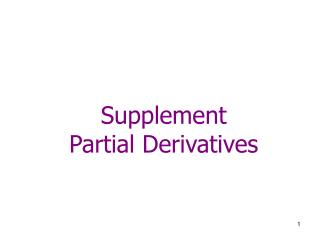DownloadDownload PresentationSupplement Partial Derivatives

# Supplement Partial Derivatives

Télécharger la présentation## Supplement Partial Derivatives

- - - - - - - - - - - - - - - - - - - - - - - - - - - E N D - - - - - - - - - - - - - - - - - - - - - - - - - - -
##### Presentation Transcript

1. SupplementPartial Derivatives

2. 1-D Derivatives (Review) Given f(x). f'(x) = df/dx = Rate of change of f at point x w.r.t. x f(x + h) – f(x) h x x+h

3. 1st-order Partial Derivatives For a function with 2 variables, f(x, y)

4. Evaluating Partial Derivatives To find fx, we differentiate f w.r.t. x and treat all other variables as constants. Example 1: f(x, y) = x3y2 = (y2)x3 To evaluate fx, y2is to be treated as a constant term. Thus fx = (y2)(3x2) = 3x2y2 Example 2: f(x, y, z) = 10xyz + sin(y)exz3 = (10yz)x + (sin(y)z3)ex To evaluate fx, (10yz) and (sin(y)z3) are to be treated as constant terms. Thus fx = 10yz + (sin(y)z3)ex

5. Exercise 1) f(x, y) = xexy • fx = • fy = 2) f(x, y, z) = sin (xy) • fx = • fy = • fz =

6. 2nd-order Partial Derivatives

7. Exercise f(x, y) = x3 + x2 y – 3xy2 + y3 • fx = • fy = • fxy = • fyx =